Doubling the Cube
Go to   Fun_Math Content Table   Three Famous Problems   Delian Problem

### Doubling the cube by Greek Mathematicians

#### Menaechmus- Conic Solution

Menaechmus (circa 380 BC - 320 BC), used parabolas and rectangular hyperbola to solve the Delian problem.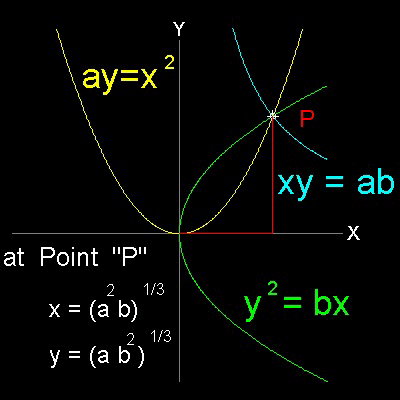``` If x, y are two mean proportional between straight lines a, b, a / x = x / y = y / b then x2 = ay, y2 = bx , and xy = ab Therefore any two pair of these three curves, will give the solution to Delian problem. (1) parabola and hyperbola-I x2 = ay, and xy = ab (2) parabola and hyperbola-II y2 = bx , and xy = ab (3) two parabolas x2 = ay, and y2 = bx ```

******** Menaechmus_Delian_desc.dwg *******

To create this drawing :
Then from command line, type Menaechmus

#### How Menaechmus drew curves of parabola and hyperbola

For a modern reader who is familiar with analytical geometry, drawing a parabola or hyperbola is not much of a task. But in the days Menaechmus lived, everything was done by lines and circles (or straight ruler and compass). Even the definition of conic curves had not been done yet. It was done about one century later by Apollonius.

#### drawing parabola    x2 = ay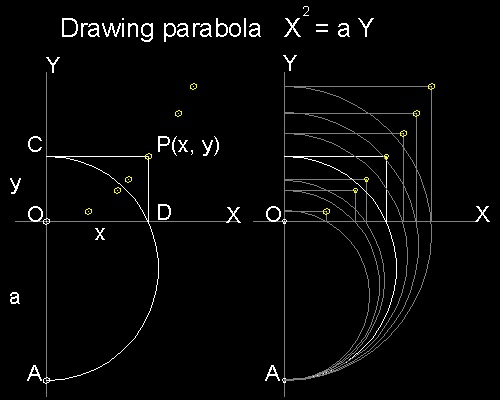AO is set to length a.
A point C is selected on y-axis.
Draw a semi-circle with AC as diameter.
This arc cuts x-axis at point D.
Then ODC, and OAD form similar triangles.
Therefore , OD2 = CO x OA, or x2 = a y
P(x,y) is a point on the parabola x2 = ay

Another form of parabola: y2 = b x
A similar argument is applicable for this type.

**************** parabola_x2_ay_desc.dwg ***************

You can see the process in animation.

To create this drawing :
Then from command line, type test_draw_x2_ay
To output jpg files for animation, use the executable auto_draw_x2_ay.

#### drawing rectangular hyperbola    xy = ab**************** hyperbola_xy_ab_desc.dwg ***************

You can see the process in animation .

The length of line segments AO and BO is set to a, and b, respectively.
Draw a vertical line CD through point B.
Draw a horizontal line CE through point A.
Select a point F on X-axis.
Drop line FG perpendicular to CE.
Draw a line from G to O, and extend it until it intersects CD at point H.
Draw a horizointal line from H.
This intersects a vertical line through F at point P
Two triangles OAG and HBO are similar.Then OA/AG = HB/OB or a/x = y/b
Therefore xy = ab
And point P (x,y) is the point on rectangular hyperbola : xy = ab

To create this drawing and animation:
Then from command line, type test_draw_xy_ab
To output jpg files for animation, use auto_draw_xy_ab after setting up jpgfile and jpg_yes flag.

#### parabola and hyperbola-I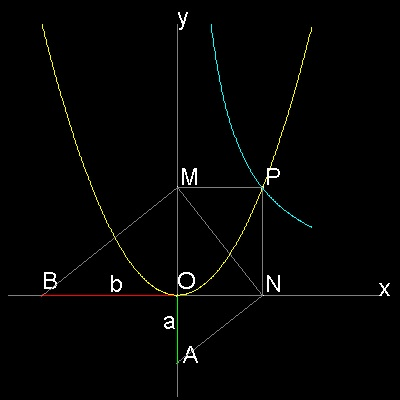In the drawing
ON = (b/a)1/3 and OM = (b/a)2/3
When a = 1, b = 2, ON = (2)1/3 and OM = (2)2/3

To create this drawing :
Then from command line, type Menaechmus_1

Note that both angles BMN and MNA are 90 degrees.(Why ?)
This observation lead the group of Plato's academy to invent the device to find the Delian problem,which will be described in the next section.

********** Menaechmus_Delian_1.dwg *********

#### parabola and hyperbola-II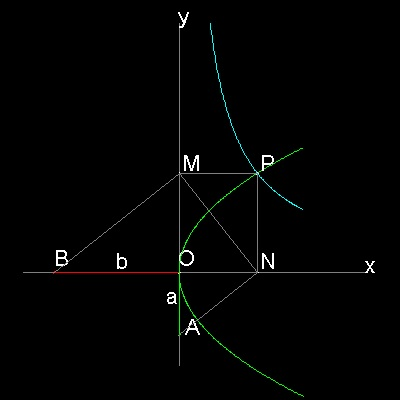To create this drawing :
Then from command line, type Menaechmus_2

********** Menaechmus_Delian_2.dwg *********

#### two parabolas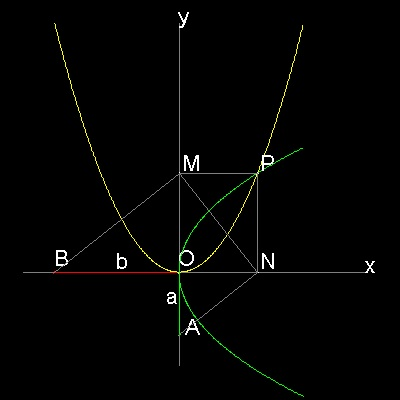To create this drawing :